Basic Electronics

# Equivalent Resistance of Complex Circuits, Series & Parallel

## Equivalent Resistance of simple and complex circuit:

So let’s say if you have two resistors R1 = 10 and R2 = 20 connected in series.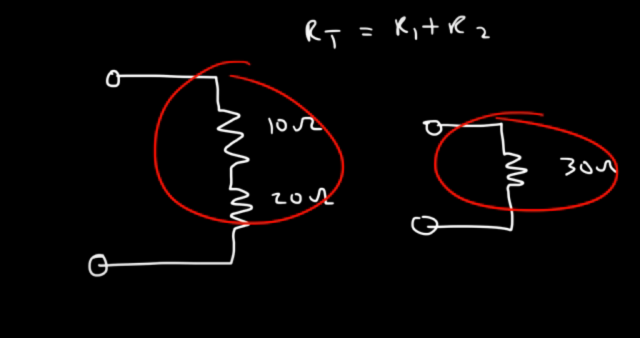let’s say this is a 10 ohm resistor and this is a 20 ohm resistor. What is the equivalent resistance or the total resistance of the circuit? In a series circuit the total resistance is simply the sum of all the resistors. So 10 + 20 is 30. So we can describe this as a 30 ohms resistor. So these two circuits are equivalent. You can simply replace the two resistors with a single resistor.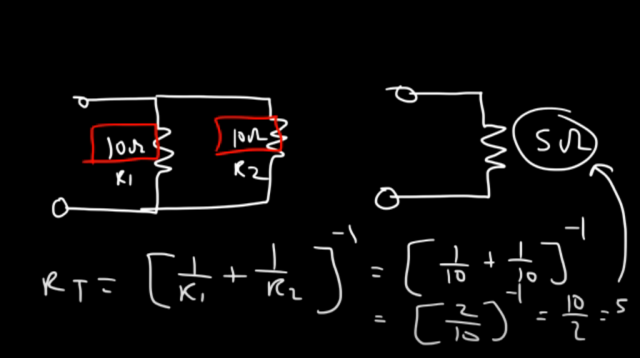Now, what if we have two resistors in parallel. Let’s say this is a 10 ohm resistor and this is a 10 ohm resistor. We’ll call these R1 and R2. So what’s the equivalent resistance of this circuit? Now whenever you have two resistors in parallel and if they have the same value the equivalent resistance will simply be half of that value, so the equivalent resistance is going to be 5 ohms. To calculate the total resistance for two resistors in a parallel circuit it’s (1/ R1 + 1/R2)-1. So this is gonna be (1 / 10 + 1 / 10 )-1 . Now 1 + 1 is 2, so this becomes 2 of a 10 and when you raise a fraction to the negative 1 power, you basically flip the fraction and so this becomes half of 10 or 10 divided by 2 which is 5.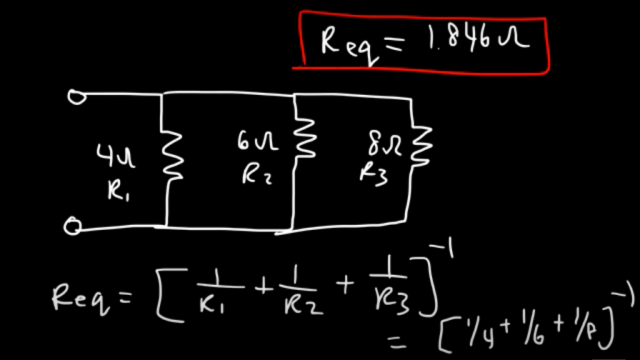Now what if we have three resistors in parallel. Let’s say this is a four ohm resistor a six ohm resistor and an 8 ohm resistor. What’s the equivalent resistance of this circuit?

Let’s call this R1, R2, and R3. So we can use this formula to get the answer it’s going to be (1/R1 + 1/R2 + 1/R3)-1. So that’s (1/ 4 + 1 / 6 + 1 / 8 )-1 and so for this circuit the equivalent resistance is 1.846 ohms and so that’s the answer.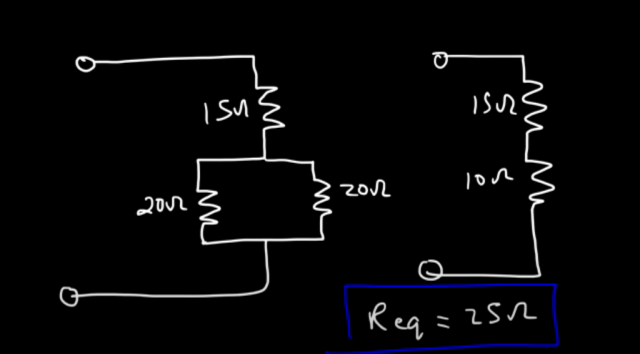Here’s another example go ahead and calculate the equivalent resistance of this circuit. So let’s say we have two 20 ohm resistors which are connected in parallel and another 15 ohms resistor. So notice that these two resistors are in parallel to each other and they have the same value so therefore the equivalent resistance of these two resistors you could replace both of them with a 10 ohm resistor. So, at this point you can replace the two resistors with a single resistor 10 ohm. Now this will make the series circuit with the 15 ohm resistor. Now the 10 ohm and 15 ohm resistors are connected in series and now what you can do is simply add the two resistances and you will get the total resistance. So 15 + 10 = 25 ohms. So the equivalent resistance for this circuit is 25 ohms and so that’s it for this problem.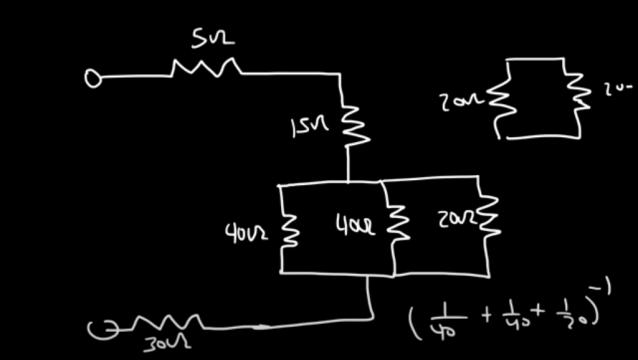So here’s another one, so let’s say three resistors 40 ohm, 40 ohm and 20 ohm are connected in parallel and there are three more resistor 5 ohm, 15 ohm and 30 ohm in the same circuit as well. As explained earlier if the two resistors are connected in parallel and they have the same values, we can simply make the value half. So as you can see the two 40ohm resistors are connected parallel so we will simple replace it with 20 ohms, another 20 ohm resistor so if these two combine is 20 and that becomes one resistor and this is 20 then these two become 10. So the equivalent resistance for all three of these resistors is 10 and to confirm it you can type it in (1/40 +  1 / 40 + 1 / 20)-1 This will give you 10 ohms.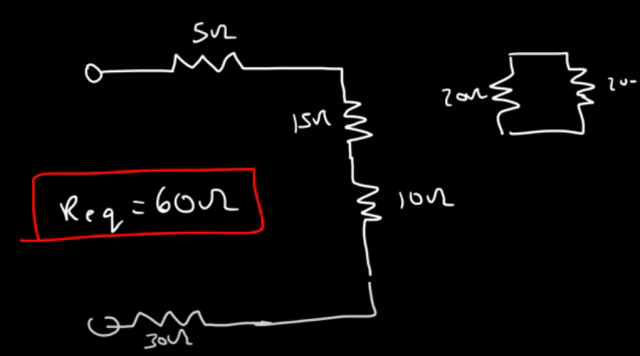So I can replace this entire parallel circuit with a 10 ohm resistor, so now the remaining four resistors notice that they’re all in series there’s only one path for the current to flow, so it’s gonna be 5 plus 15 which is 20 plus 10 plus 30, so the equivalent resistance for this circuit is 60 ohms and that’s it for this problem.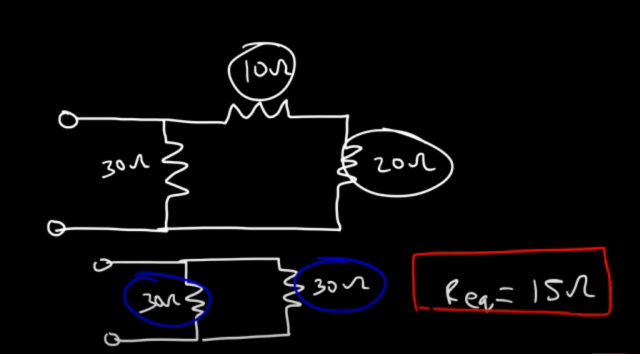Let’s try this example. so let’s say this is a 10 ohm resistor this is 20 and this is 30, so feel free to stop over here and calculate the equivalent resistance of the circuit. So notice that these two they’re in series because there’s only one path for the current to flow so 10 + 20 is 30 ohms. so I can replace those two resistors with a 30m resistor now I can write this resistor like this shown at the bottom, or if you draw it some other way, it doesn’t matter what’s important is to realize that these two when you combine it into a single resistor they’re in parallel with the other 30 ohm resistor, so once you realize that and the fact that you have two parallel resistors with the same value you know that the equivalent resistance is going to be half of 30 so it’s 15 ohms and that’s the answer.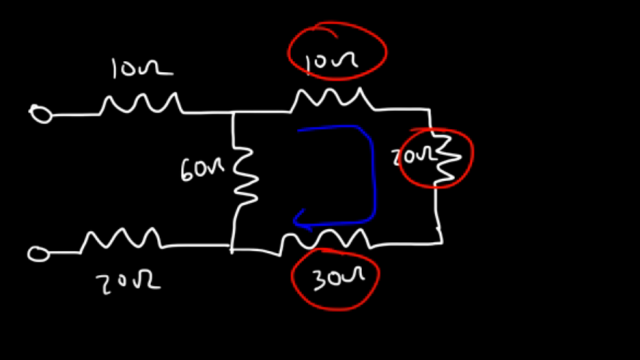Now let’s try a similar example but with more steps. Resistors encircled Red, if this is 10 this is 20 and it’s just 30 we’re gonna say that this is 60. First we need to realize that these three resistors are in serious there’s only one path for the current to flow in that branch so we can add 10, 20, and 30 to get 60 so let’s replace this with a 60 own resistor. So, when you replace all the three resistors with a single 60 ohm resistor, you will find that this resistor is going to be in parallel with the 60 ohm resistor. So same value resistors connected in parallel, and you know if the resistors are of the same value, simply half the value, so it will be 30 ohms.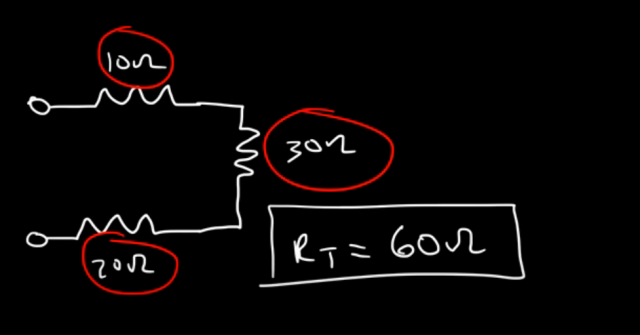Now we have three resistors in series 10, 30 and 20 that gives us an equivalent resistance or total resistance of 60 ohms for that circuit.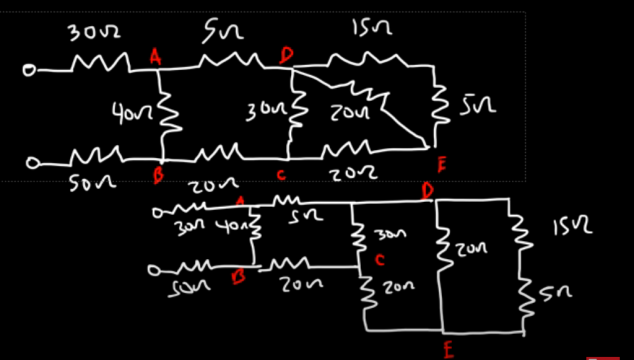Let’s look at one more example. This one’s gonna be harder than the other ones but you should definitely try. There are too many resistors connected in parallel and series combination. Whenever you try to solve a circuit like the one you can see on the screen, first of all, at a look at the circuit and try to understand which resistors are connected in series and which resistors are connected in parallel. If Still you find it hard to understand, you can redraw the circuit to better understand the connections. In the image above you can see the other circuit is the simplified version of the original circuit, which is quite easy to understand.

So on the right side of the circuit you can see 15 ohm and 5 ohm resistors are connected in series so go ahead and solve, 15 + 5 = 20. You can replace these two resistors with a single resistor of 20 ohms.  Now, you can redraw the circuit.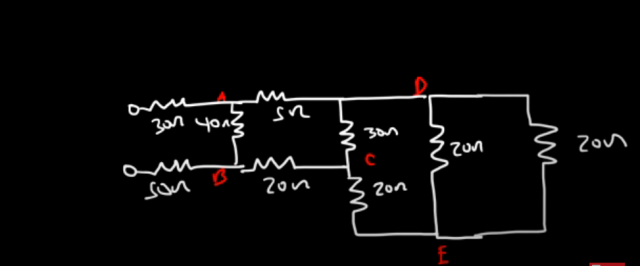Now you can see the two resistors are of the same value and are connected in parallel, You know if two resistors are connected in parallel and they have the same values then simply divide the value by 2, or in simple words simply half the value which is going to be 10 ohm. Now you can further redraw the circuit and replace the two resistors with a single resistor of 10 ohms between the points D and E.

Now you will find that the 10 ohm resistor is in series with the 20 ohm resistors. You can add the two resistors and you will get 30 ohms.

Now, further you will find that the 30 ohm resistor is now in parallel with the other 30 ohm resistor. As the resistors are same and are in parallel so half the value and you will get 15 ohms.

Now you will find that the resistor 5, 15, and 20 are in series. So go ahead and add them 5+15+20 = 40.

Now this resistor which is 40 ohms will now be in parallel with the resistor connected between the points A and B. So as both the resistors are connected in parallel and are of the same value so we will simply half the value. So we will get 20 ohms. Now you can redraw the circuit.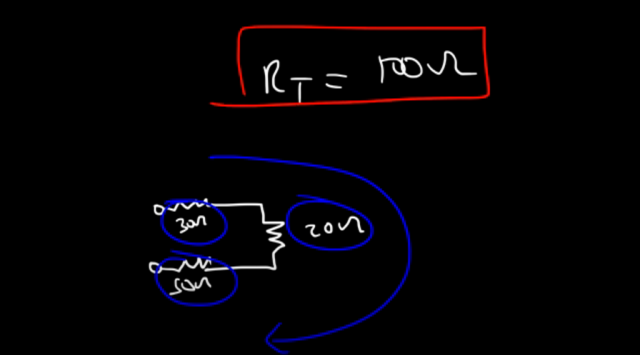Now all the three resistors are connected in series, and now you know, what you have to do, is just add these resistors. So, 30 + 20 + 50 = 100 ohms.